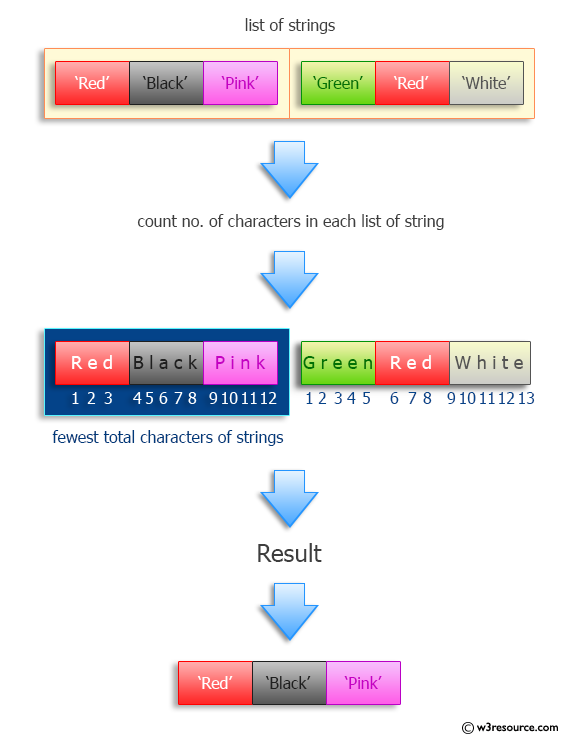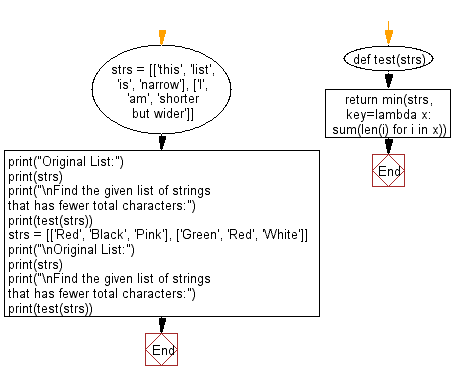﻿ Python: Find the list that has fewer total characters (including repetitions) - w3resource# Python: Find the list that has fewer total characters (including repetitions)

## Python Programming Puzzles: Exercise-30 with Solution

Write a Python program to find the list of strings that has fewer total characters (including repetitions).

```Input:
[['this', 'list', 'is', 'narrow'], ['I', 'am', 'shorter but wider']]

Output:
['this', 'list', 'is', 'narrow']

Input:
[['Red', 'Black', 'Pink'], ['Green', 'Red', 'White']]

Output:
['Red', 'Black', 'Pink']
```

Pictorial Presentation:Sample Solution:

Python Code:

``````#License: https://bit.ly/3oLErEI

def test(strs):
return min(strs, key=lambda x: sum(len(i) for i in x))
strs = [['this', 'list', 'is', 'narrow'], ['I', 'am', 'shorter but wider']]
print("Original List:")
print(strs)
print("\nFind the given list of strings that has fewer total characters:")
print(test(strs))
strs = [['Red', 'Black', 'Pink'], ['Green', 'Red', 'White']]
print("\nOriginal List:")
print(strs)
print("\nFind the given list of strings that has fewer total characters:")
print(test(strs))
``````

Sample Output:

```Original List:
[['this', 'list', 'is', 'narrow'], ['I', 'am', 'shorter but wider']]

Find the given list of strings that has fewer total characters:
['this', 'list', 'is', 'narrow']

Original List:
[['Red', 'Black', 'Pink'], ['Green', 'Red', 'White']]

Find the given list of strings that has fewer total characters:
['Red', 'Black', 'Pink']
```

Flowchart:## Visualize Python code execution:

The following tool visualize what the computer is doing step-by-step as it executes the said program:

Python Code Editor :

Have another way to solve this solution? Contribute your code (and comments) through Disqus.

What is the difficulty level of this exercise?

Test your Programming skills with w3resource's quiz.

﻿

## Python: Tips of the Day

Clamps num within the inclusive range specified by the boundary values x and y:

Example:

```def tips_clamp_num(num,x,y):
return max(min(num, max(x, y)), min(x, y))
print(tips_clamp_num(2, 4, 6))
print(tips_clamp_num(1, -1, -6))
```

Output:

```4
-1
```